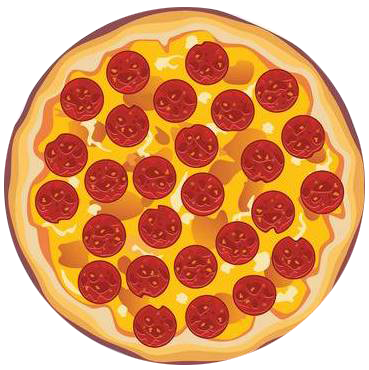IntroductionIntroduction# Improper Fractions and Mixed Numbers

To help us better understand mixed numbers and improper fractions, we like to imagine them as pizzas or legos.

Pick whichever you vibe with more:

INTRO
A mixed number tells us how many whole numbers and parts we have, and an improper fraction tells us how many total parts we have when the parts make up at least one whole.
We can use an improper fraction and a mixed number to represent the same thing. For example, we can either say we have 2 whole pizzas and 3 slices of pizza, where 4 slices make up a whole:Or we can say we have slices of pizza where 4 slices make up a whole:They're just two ways of saying the same thing 👯.
Check out our
Calculator
or explore our
Lesson
and
Practice

CALCULATOR

Convert a

KEY STEPS

## How to Convert from Improper Fractions to Mixed Numbers

Convert a

### Step 1. Explain the fraction.

In pizza terms, for example, means there are total slices where slices make up a whole pizza.

### Step 2. Set up the conversion.

We want to split up our fraction into a whole number and the leftover fraction:
In pizza terms, this is like figuring out how many whole pizzas and leftover slices there are:

### Step 3. Find the whole number.

This is equal to the number of times the denominator goes into the numerator and tells us how many whole numbers we can make from the total number of equal parts we have.
In pizza terms, we're figuring out how many whole pizzas we can make:

### Step 4. Find the leftover/remainder.

In pizza terms, this is like finding the leftover slices after forming our whole pizzas:
Ex:

### Step 5. Drop the plus sign.

Ex:
LESSON
Converting Improper Fractions to Mixed Numbers

## Converting Improper Fractions to Mixed Numbers

An improper fraction is a fraction where the numerator is greater than or equal to the denominator. It tells us how many equal-sized parts there are and how big the parts are.

To convert an improper fraction into a mixed number, we have to figure out how many wholes can be created from the parts, and then how many leftover parts are left.
So, if we have slices where slices make up a whole pizza, then we can make whole pizza, with slices leftover:

### Improper Fraction### Mixed NumberLet's try some examples!
PRACTICE
Converting Improper Fractions to Mixed Numbers

## Practice: Converting Improper Fractions to Mixed Numbers

Question 1 of 10: Convert into a mixed number.

### Step 1. Explain the fraction.

In pizza terms, for example, means there are total slices where slices make up a whole pizza.

LESSON
Converting Mixed Numbers to Improper Fractions

## Converting Mixed Numbers to Improper Fractions

A mixed number is a combination of a whole number and a fraction. It tells us how many whole numbers there are and many leftover parts there are.

To convert a mixed number into an improper fraction, we have to figure out how many parts are in the wholes, and then add that to the leftover parts to get the total number of equal-sized parts.
If we have whole pizzas where slices make up a whole pizza, then we have slices. Adding that to the slices leftover, we have a total of slices:

### Mixed Number### Improper FractionLet's try some examples!
PRACTICE
Converting Mixed Numbers to Improper Fractions

## Practice: Converting Mixed Numbers to Improper Fractions

Question 1 of 10: Convert into an improper fraction.

### Step 1. Add the plus sign.

Add in the hidden plus sign between the whole number and fraction.

CONCLUSION
Incredible job, look at you go! Thanks for checking out this lesson ☺️🙏. Where to next?Leave Feedback